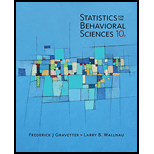Chapter 3, Problem 2PStatistics for The Behavioral Scie...

10th Edition
Frederick J Gravetter + 1 other
ISBN: 9781305504912

Solutions

Chapter
SectionStatistics for The Behavioral Scie...

10th Edition
Frederick J Gravetter + 1 other
ISBN: 9781305504912
Textbook Problem

Find the mean, median, and mode for the following sample of scores: 6, 7, 3, 9, 8, 3, 7, 5

To determine

Find the mean, median and mode for the given sample of scores.

Explanation

Given:

The sample of scores:

3, 6, 7, 3, 9, 8, 3, 7, 5

Here n=9 is odd.

Formula used:

M=xn

Median=(n+12)thscore

Calculations:

To find mean, add all scores and divide the sum by number of scores in the sample.

M=xn=3+6+7+3+9+8+3+7+59=519=5.67

To find median, first arrange data in ascending order as shown below and then find the middle score.

 3 3 3 5 6 7 7 8 9

For nine scores, the median would be (n+12)th=(9+12)th=5th score of the sorted data.

Median=(n+12)thscore=5thscore=6

The mode is most occurred score in the sample

Still sussing out bartleby?

Check out a sample textbook solution.

See a sample solution

The Solution to Your Study Problems

Bartleby provides explanations to thousands of textbook problems written by our experts, many with advanced degrees!

Get Started

Is there a number that is exactly 1 more than its cube?

Single Variable Calculus: Early Transcendentals, Volume I

39. Find the average rate of change of

Mathematical Applications for the Management, Life, and Social Sciences

Study Guide for Stewart's Multivariable Calculus, 8th

Sometimes, Always, or Never: If 0 ≤ an ≤ bn for all n and diverges, then converges.

Study Guide for Stewart's Single Variable Calculus: Early Transcendentals, 8th